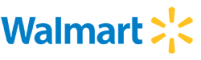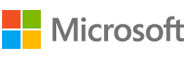New update is available. Click here to update.
Topics

# Deepest Leaves Sum

Easy0/40
Average time to solve is 15m+1 more companies

## Problem statement

You have been given a binary tree of integers. Your task is to calculate the sum of all the leaf nodes which are present at the deepest level of this binary tree. If there are no such nodes, print 0.

NOTE: The deepest level of a binary tree is the level which is present at the maximum depth from the root node.

``````Can you solve this problem in a single traversal of the binary tree?
``````
Detailed explanation ( Input/output format, Notes, Images )
Constraints:
``````1 <= T <= 10^2
0 <= N <= 3000
Where N is the number of nodes in the binary tree

The sum will always fit in a 32-bit integer.

Time Limit: 1 sec
``````
Sample Input 1:
``````3
71 2 16 110 -1 -1 5 -1 -1 -1 -1
1 2 3 4 5 6 7 -1 -1 -1 -1 -1 -1 -1 -1
-1
``````
Sample Output 1:
``````115
22
0
``````
Explanation of Sample Input 1:
``````For the first test case, we have nodes 110 and 5 present at the deepest level of the binary tree. So the sum will be 110 + 5 = 115.

For the second test case, we have nodes 4, 5, 6 and 7 present at the deepest level of the binary tree.

For the third test case, we don't have any such nodes. So the sum will be 0.
``````
Sample Input 2:
``````2
1 2 3 4 -1 5 -1 -1 -1 -1 -1
1 2 -1 3 -1 4 -1 -1 -1
``````
Sample Output 2:
``````9
4
``````Console